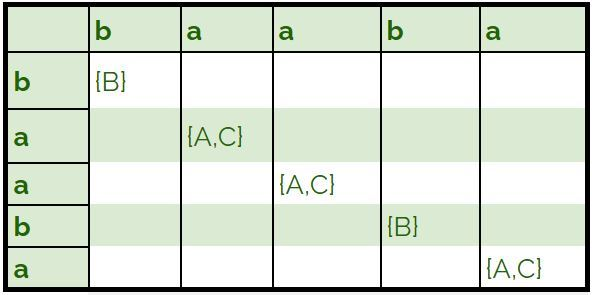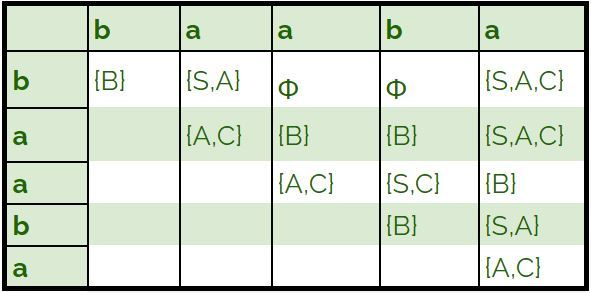# CYK Algorithm for Context Free Grammar

Prerequisite – Converting Context Free Grammar to Chomsky Normal Form
CYK algorithm is a parsing algorithm for context free grammar.
In order to apply CYK algorithm to a grammar, it must be in Chomsky Normal Form. It uses a dynamic programming algorithm to tell whether a string is in the language of a grammar.

Algorithm :
Let w be the n length string to be parsed. And G represent the set of rules in our grammar with start state S.

1. Construct a table DP for size n × n.
2. If w = e (empty string) and S -> e is a rule in G then we accept the string else we reject.
3. ```For i = 1 to n:
For each variable A:
We check if A -> b is a rule and b = wi for some i:
If so, we place A in cell (i, i) of our table. ```
4. ```For l = 2 to n:
For i = 1 to n-l+1:
j = i+l-1
For k = i to j-1:
For each rule A -> BC:
We check if (i, k) cell contains B and (k + 1, j) cell contains C:
If so, we put A in cell (i, j) of our table. ```
5. ```We check if S is in (1, n):
If so, we accept the string
Else, we reject.```

Example –
Let our grammar G be:

```S -> AB | BC
A -> BA | a
B -> CC | b
C -> AB | a ```

We check if baaba is in L(G):

1. We first insert single length rules into our table.2. We then fill the remaining cells of our table.3. We observe that S is in the cell (1, 5), Hence, the string baaba belongs to L(G).

Time and Space Complexity :

• Time Complexity –
`O(n3.|G|) `

Where |G| is the number of rules in the given grammar.

• Space Complexity –
`O(n2)  `
Whether you're preparing for your first job interview or aiming to upskill in this ever-evolving tech landscape, GeeksforGeeks Courses are your key to success. We provide top-quality content at affordable prices, all geared towards accelerating your growth in a time-bound manner. Join the millions we've already empowered, and we're here to do the same for you. Don't miss out - check it out now!

Previous
Next